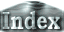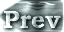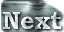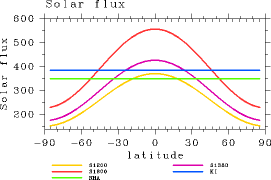# 2. Model and Experimental Design### c. Experimental Design and List of Experiments

The values listed in Table 1 are given as the solar constants S . For each case, integration is carried out for 1000 days or longer. (SSR is global mean value of incident shortwave radiation flux and SSR = S/4.)

The planet is assumed to have the same orbital parameters as the present Earth, and calculations will be performed by supplying the annually and daily mean incident solar radiation flux distribution, which is known as the perpetual annual mean condition. Based on these conditions, only the solar constant is varied virtually. Therefore, the meridional distribution of solar flux will take the form shown in Fig. 1. While it would be interesting to see the results for cases where the solar radiation distribution is changed, or when diurnal variations are considered, such cases are beyond the scope of the present study.

The initial conditions of all calculations in the present study are the same: a resting, globally isothermal atmosphere (280 K) with constant specific humidity (10-5).

The initial surface temperature distribution has zonal symmetry, with high and low temperatures in the equatorial and polar regions, respectively.

 Experimet name S (W/m2) SSR (W/m2) Note S1200 1200 300.0 S1380 1380 345.0 the present Earth condtion S1500 1500 375.0 S1550 1550 387.5 S1570 1570 392.5 S1600 1600 400.0 runaway state S1700 1700 425.0 runaway state S1800 1800 450.0 runaway state

Table1: Values of solar constats given in each experimets.Figure 1: Meridional distributions of incoming solar radiation (W/m2). The red, purple, and yellow lines show in the case of S=1800 W/m2, S=1380 W/m2, S=1200 W/m2, respectively. The blue line shows Komabayashi-Ingersoll limit (385.2 W/m2), and the green line shows the upper limit of outgoing longwave radiation (355.0 W/m2) calculated by using a 1D radiative convective equilibrium model (Nakajima et al., 1992).

 2.c. Experimental Design and List of Experiments